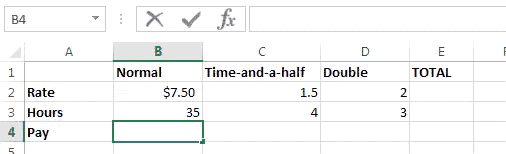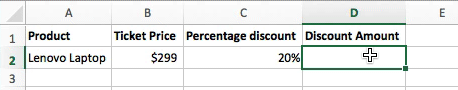### Excel formula

#### Net Pay | # gomaths.net/4216

Calculate net pay following deductions from gross pay.

`=B1-B2`
where `B1` is gross pay and `B2` is the deductions#### Overtime | # gomaths.net/4219

Calculate pay for normal hours, time-and-a-half and double-time.#### Percentage discount | # gomaths.net/4785

Calculate a percentage discount.

`=B2-C2*B2`